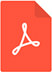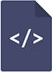# Direct applications of homotopy perturbation method for solving nonlinear algebraic equationsPDF Full Text

# Abstract

In this work, homotopy perturbation method is directly applied to provide solutions to nonlinear algebraic equations. The reliability and efficiency of the method in solving nonlinear algebraic equations are demonstrated through different illustrative numerical examples. The method is shown to be conceptually and computationally simple and straightforward without any ambiguity. Also, the superiority of the direct applications of the approximate analytical method over the other methods show that it does not require the development of any other iterative scheme that could be used to find the solution of the nonlinear equations. With the use of the homotopy perturbation method, there is no search for an auxiliary parameter for adjusting and controlling the rate and region of convergence of the solution.Additionally, the approximate analytical method does not entail the determination of Adomian polynomials and finding symbolic or numerical derivatives of any given function. The method does not require finding the correct fixed point and it is free from the problem of choosing an appropriate initial approximation. Therefore, it is hoped that the present work will assistin providing accurate solutions to many practical problems in science and engineering.

# Keywords

nonlinear algebraic equations, root-finding method; homotopy perturbation method